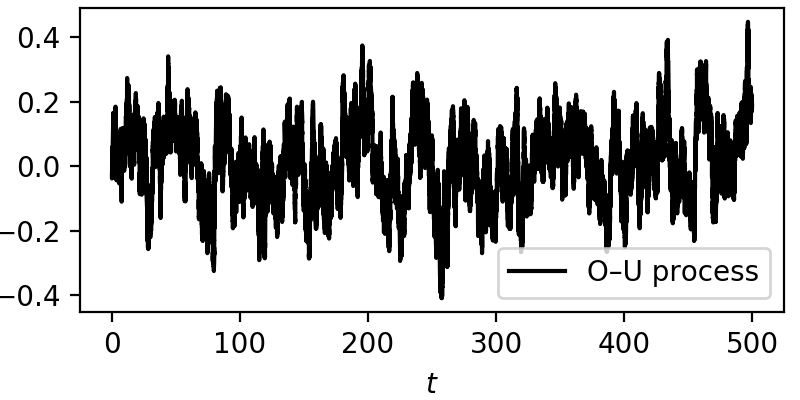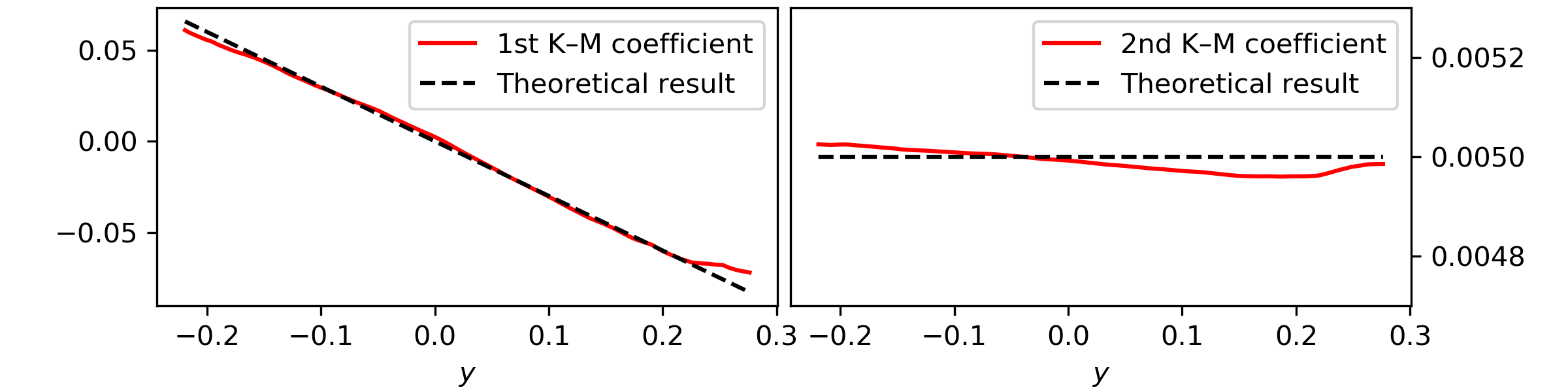# A one-dimensional stochastic process¶

## The theory¶

Take, for example, the well-documented one-dimension Ornstein—Uhlenbeck process, also known as Vašíček process. This process is governed by two main parameters: the mean-reverting parameter $$\theta$$ and the diffusion or volatility coefficient $$\sigma$$

$\mathrm{d}y(t) = -\theta y(t)\mathrm{d}t + \sigma \mathrm{d}W(t)$

which can be solved in various ways. For our purposes, recall that the drift coefficient, i.e., the first-order Kramers—Moyal coefficient, is given by $$\mathcal{M}^{}(y) = -\theta y$$ and the second-order Kramers—Moyal coefficient is $$\mathcal{M}^{}(y) = \sigma^2 / 2$$, i.e., the diffusion.

For this example let’s take $$\theta=0.3$$ and $$\sigma=0.1$$, over a total time of 500 units, with a sampling of 1000 Hertz, and from the generated data series retrieve the two parameters, the drift $$-\theta y(t)$$ and diffusion $$\sigma$$.

## Integrating an Ornstein—Uhlenbeck process¶

Here is a short code on generating a Ornstein—Uhlenbeck stochastic trajectory with a simple Euler–Maruyama integration method

# integration time and time sampling
t_final = 500
delta_t = 0.001

# The parameters theta and sigma
theta = 0.3
sigma = 0.1

# The time array of the trajectory
time = np.arange(0, t_final, delta_t)

# Initialise the array y
y = np.zeros(time.size)

# Generate a Wiener process
dw = np.random.normal(loc = 0, scale = np.sqrt(delta_t), size = time.size)

# Integrate the process
for i in range(1,time.size):
y[i] = y[i-1] - theta*y[i-1]*delta_t + sigma*dw[i]


From here we have a plain example of an Ornstein—Uhlenbeck process, always drifting back to zero, due to the mean-reverting drift $$-\theta y(t)$$. The effect of the noise can be seen across the whole trajectory.## Using kramersmoyal¶

Take the timeseries $$y(t)$$ and let’s study the Kramers—Moyal coefficients. For this let’s look at the drift and diffusion coefficients of the process, i.e., the first and second Kramers—Moyal coefficients, with an epanechnikov kernel

# Choose number of points of you target space
bins = np.array()

# Choose powers to calculate
powers = np.array([, ])Notice here that to obtain the Kramers—Moyal coefficients you need to divide kmc by the timestep delta_t. This normalisation stems from the Taylor-like approximation, i.e., the Kramers—Moyal expansion ($$\delta t \to 0$$).